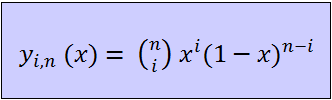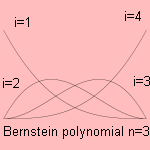# Bernstein polynomial

## polynomialThe Bernstein polynomial Bi, n (x) has some useful properties: normalization between 0 and 1 single unique local maximum at x=i/n positiveThe polynomial occurred as result of the work of Sergei Natanovich Bernstein, an Ukrainian mathematician (1880-1968). Bernstein introduced the curve in 1911, using it for a constructive proof for the Stone-Weierstrass approximation theorem. Paul de Faget de Casteljau used the polynomials for the approximation of curves, for the French car company Citroën. Working for Citroëns competitor Renault, Pierre Bézier (1910-1999) came to the same curves, which he mentioned Bézier curves. His initial idea was to construct a basic curve as the intersection of two elliptic cylinders, later he moved to polynomials. It was AR. Forrest who realized that Faget de Casteljau and Bézier did the same job Therefore we call the Bézier curve also the Bernstein-Bézier curve. It can be expressed as Bernstein polynomials Bi,n, given a set of n+1 control points Pi (Pix, Piy):The curve has the following properties: there is no line that has more intersections with a Bézier curve than with the curve composed by the line segments through the points. the curve can be translated and rotated by performing these operations on the control points. a numerical instability for large numbers of control points. moving a single control point changes the global shape of the curve. This can be avoided by smoothly patching together low-order Bézier curves. Another generalization of the curve is the B-spline.